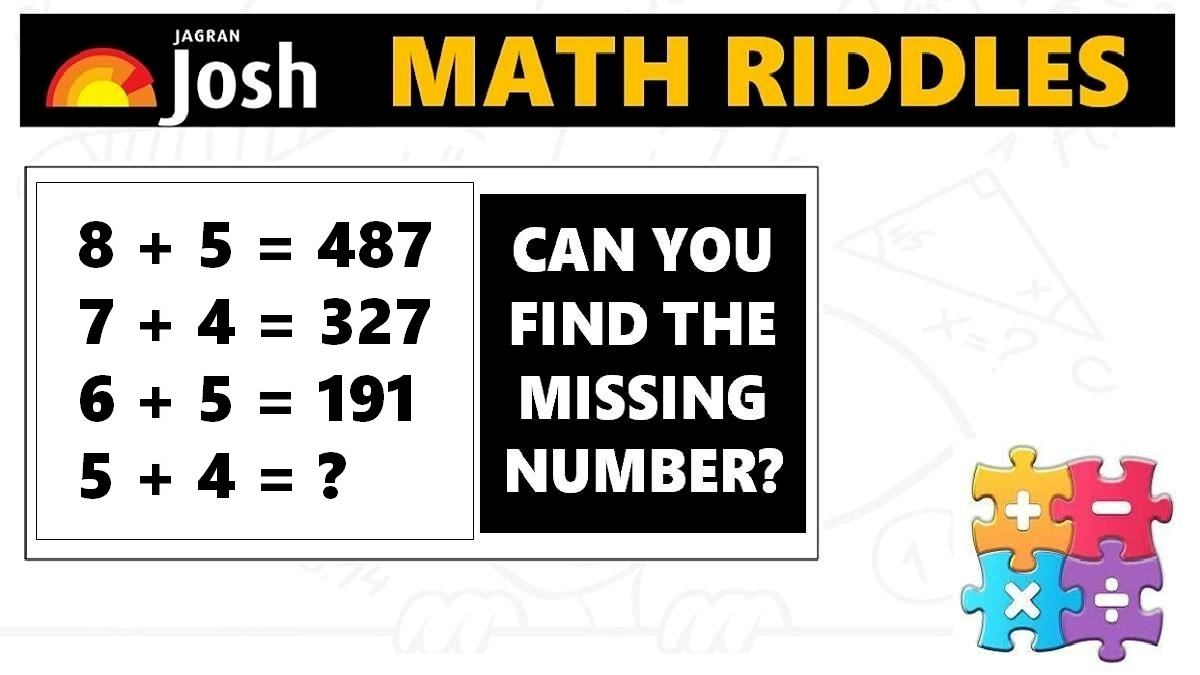Maths Puzzles with Answers: Check out these math riddles for geniuses. Find out the missing numbers for each puzzle in less than 1 minute. Check solutions to learn easy way to solve math puzzles.Math Riddles: Check out these math riddles for geniuses. Find out the missing numbers for each puzzle in less than 1 minute. Check solutions to learn easy way to solve math puzzles. Plus, these maths concepts will help you in acing your preparation for quantitative aptitude, reasoning ability, arithmetic problems, etc in competitive exams such as banking, civil services, defence, etc.

Explanation:

Explanation:

## Tell us in comments: Did you solve these mathematics puzzles in less than 1 minutes each?

Check out more math puzzles!

Also Read: Math Riddles: Only 1% genius can solve these mathematics puzzles

Also Read: Math Riddles: Only High IQ Genius Can Solve These

Also Read: Math Riddles: Check Your Brain Power, Solve these Mathematics Puzzles

Also Read: Math Riddles: Can You Find The Next Number In These Math Puzzle Series?

Get the latest General Knowledge and Current Affairs from all over India and world for all competitive exams.
खेलें हर किस्म के रोमांच से भरपूर गेम्स सिर्फ़ जागरण प्ले पर